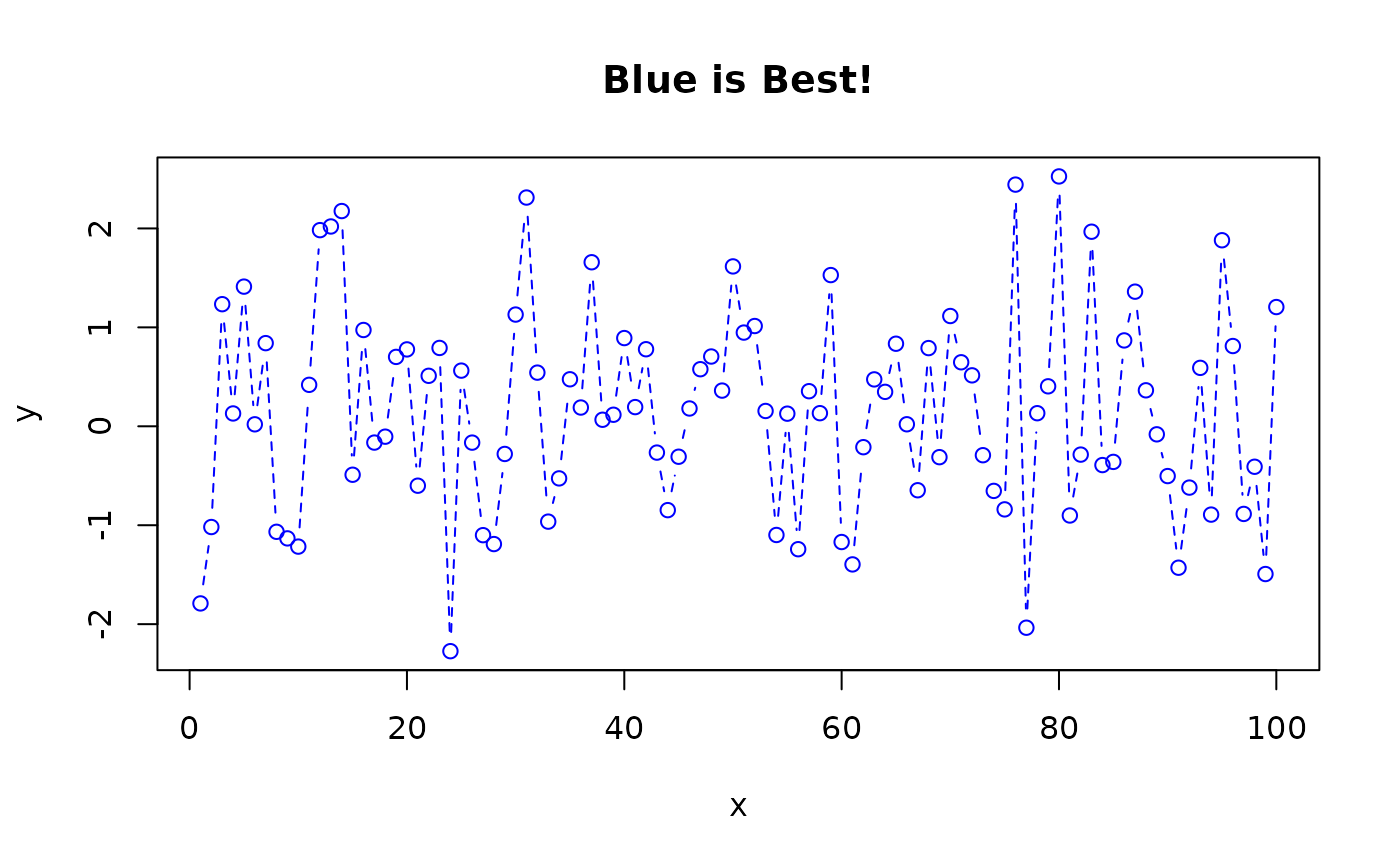defmacro define a macro that uses R expression replacement

defmacro(..., expr)

strmacro(..., expr, strexpr)

## Arguments

...

macro argument list

expr

R expression defining the macro body

strexpr

character string defining the macro body

## Value

A macro function.

## Details

strmacro define a macro that uses string replacement

defmacro and strmacro create a macro from the expression given in expr, with formal arguments given by the other elements of the argument list.

A macro is similar to a function definition except for handling of formal arguments. In a function, formal arguments are simply variables that contains the result of evaluating the expressions provided to the function call. In contrast, macros actually modify the macro body by replacing each formal argument by the expression (defmacro) or string (strmacro) provided to the macro call.

For defmacro, the special argument name DOTS will be replaced by ... in the formal argument list of the macro so that ... in the body of the expression can be used to obtain any additional arguments passed to the macro. For strmacro you can mimic this behavior providing a DOTS="" argument. This is illustrated by the last example below.

Macros are often useful for creating new functions during code execution.

## Note

Note that because [the defmacro code] works on the parsed expression, not on a text string, defmacro avoids some of the problems of traditional string substitution macros such as strmacro and the C preprocessor macros. For example, in

 mul <- defmacro(a, b, expr={a*b}) 

a C programmer might expect mul(i, j + k) to expand (incorrectly) to i*j + k. In fact it expands correctly, to the equivalent of i*(j + k).

For a discussion of the differences between functions and macros, please Thomas Lumley's R-News article (reference below).

function substitute, eval, parse, source, parse,

## Author

Thomas Lumley wrote defmacro. Gregory R. Warnes greg@warnes.net enhanced it and created strmacro.

## Examples


####
# macro for replacing a specified missing value indicator with NA
# within a dataframe
###
setNA <- defmacro(df, var, values,
expr = {
df$var[df$var %in% values] <- NA
}
)

# create example data using 999 as a missing value indicator
d <- data.frame(
Grp = c("Trt", "Ctl", "Ctl", "Trt", "Ctl", "Ctl", "Trt", "Ctl", "Trt", "Ctl"),
V1 = c(1, 2, 3, 4, 5, 6, 999, 8, 9, 10),
V2 = c(1, 1, 1, 1, 1, 2, 999, 2, 999, 999),
stringsAsFactors = TRUE
)
d
#>    Grp  V1  V2
#> 1  Trt   1   1
#> 2  Ctl   2   1
#> 3  Ctl   3   1
#> 4  Trt   4   1
#> 5  Ctl   5   1
#> 6  Ctl   6   2
#> 7  Trt 999 999
#> 8  Ctl   8   2
#> 9  Trt   9 999
#> 10 Ctl  10 999

# Try it out
setNA(d, V1, 999)
setNA(d, V2, 999)
d
#>    Grp V1 V2
#> 1  Trt  1  1
#> 2  Ctl  2  1
#> 3  Ctl  3  1
#> 4  Trt  4  1
#> 5  Ctl  5  1
#> 6  Ctl  6  2
#> 7  Trt NA NA
#> 8  Ctl  8  2
#> 9  Trt  9 NA
#> 10 Ctl 10 NA

###
# Expression macro
###
plot.d <- defmacro(df, var, DOTS,
col = "red", title = "", expr =
plot(df$var ~ df$Grp, type = "b", col = col, main = title, ...)
)

plot.d(d, V1)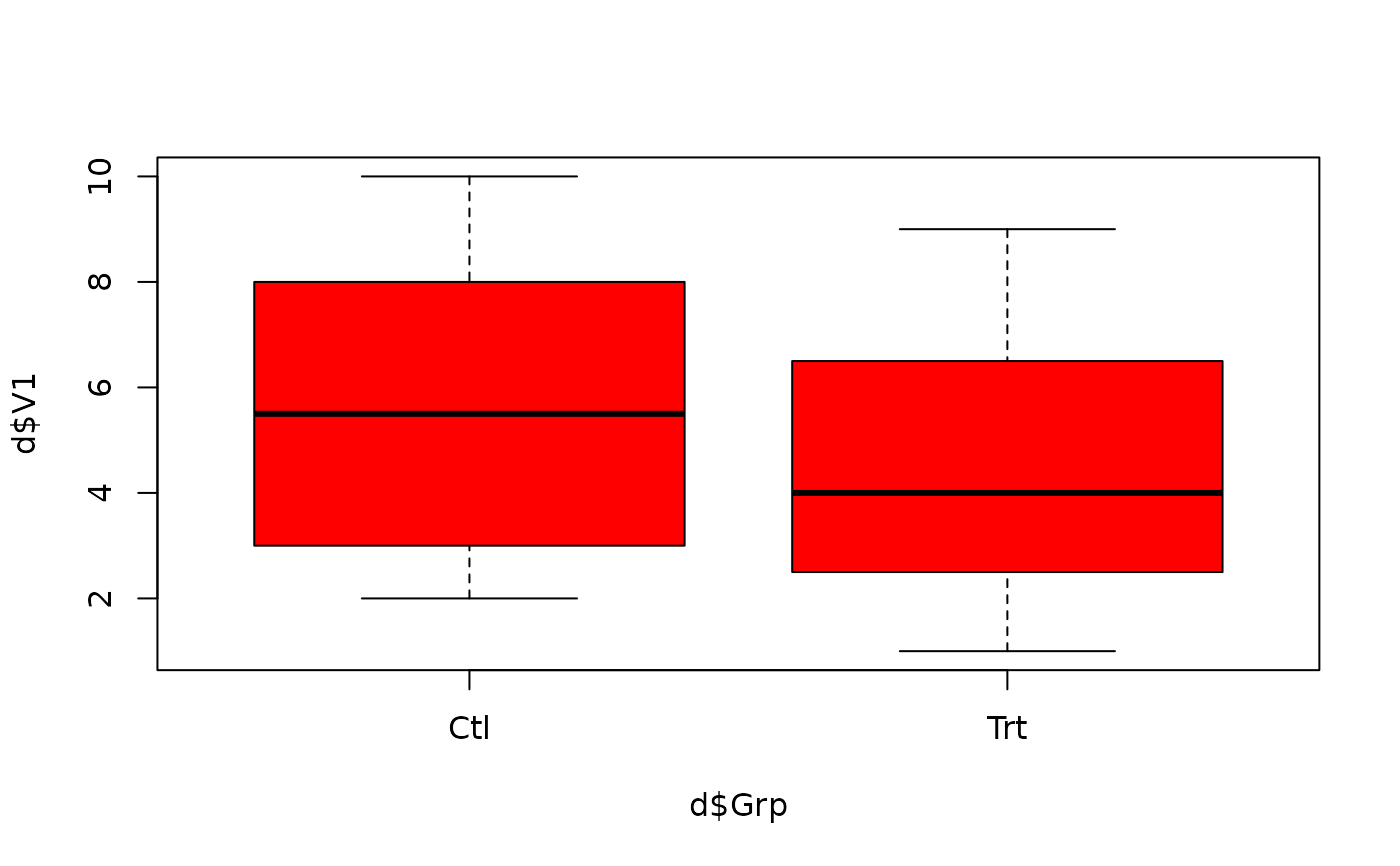plot.d(d, V1, col = "blue")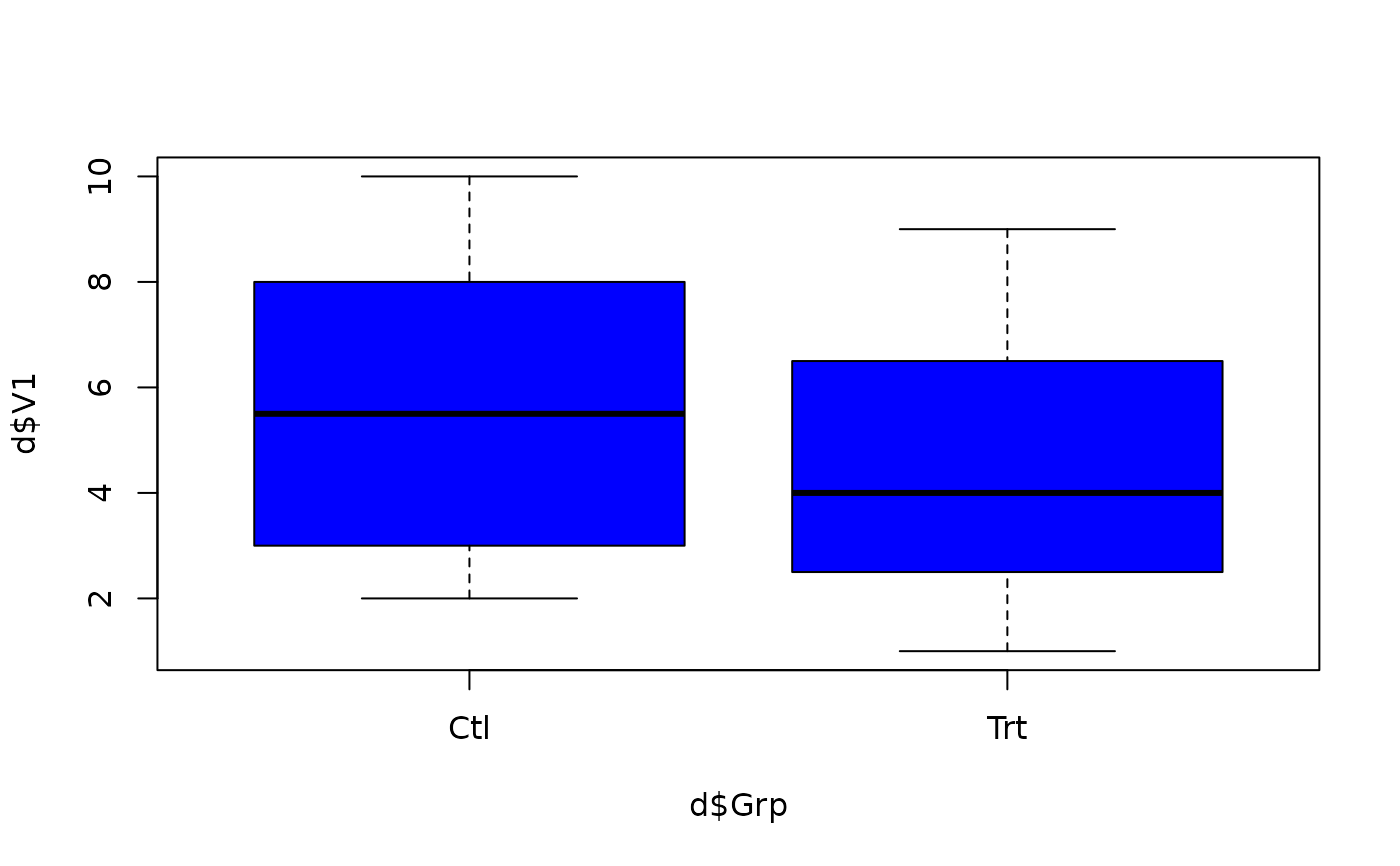plot.d(d, V1, lwd = 4) # use optional 'DOTS' argument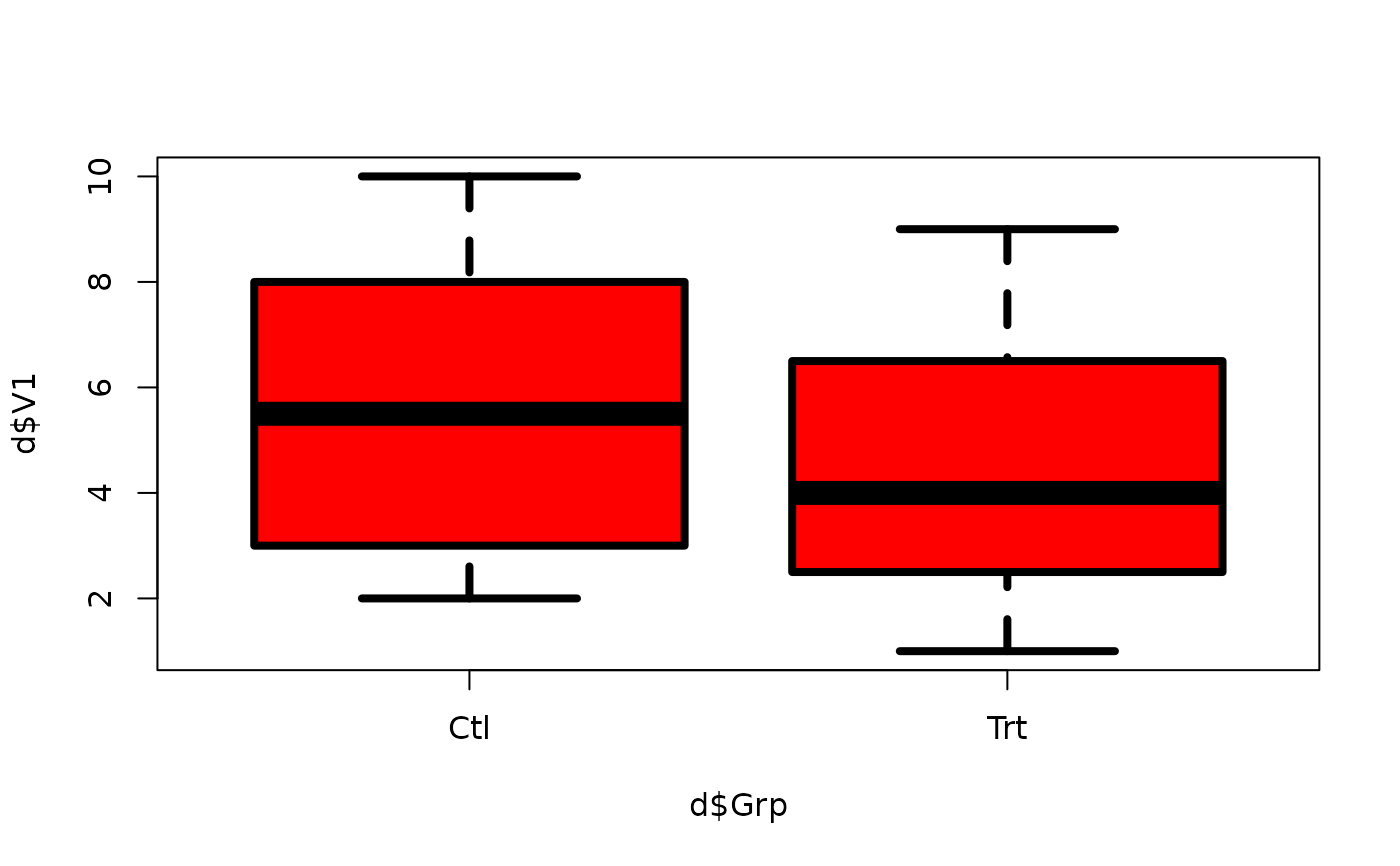###
# String macro (note the quoted text in the calls below)
#
# This style of macro can be useful when you are reading
# function arguments from a text file
###
plot.s <- strmacro(DF, VAR,
COL = "'red'", TITLE = "''", DOTS = "", expr =
plot(DF$VAR ~ DF$Grp, type = "b", col = COL, main = TITLE, DOTS)
)

plot.s("d", "V1")plot.s(DF = "d", VAR = "V1", COL = '"blue"')plot.s("d", "V1", DOTS = "lwd=4") # use optional 'DOTS' argument#######
# Create a macro that defines new functions
######
plot.sf <- defmacro(
type = "b", col = "black",
title = deparse(substitute(x)), DOTS, expr =
function(x, y) plot(x, y, type = type, col = col, main = title, ...)
)

plot.red <- plot.sf(col = "red", title = "Red is more Fun!")
plot.blue <- plot.sf(col = "blue", title = "Blue is Best!", lty = 2)

plot.red(1:100, rnorm(100))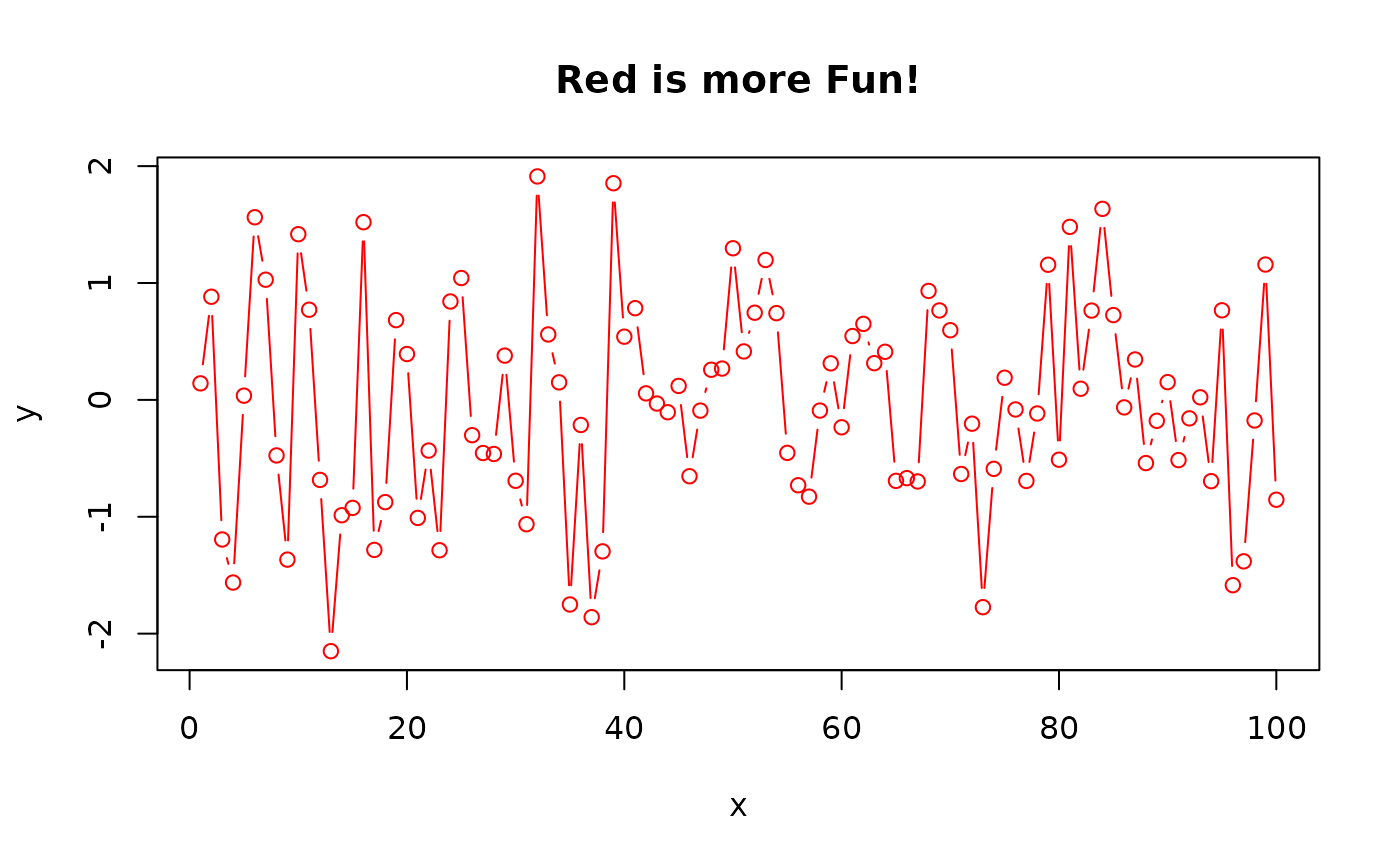plot.blue(1:100, rnorm(100))